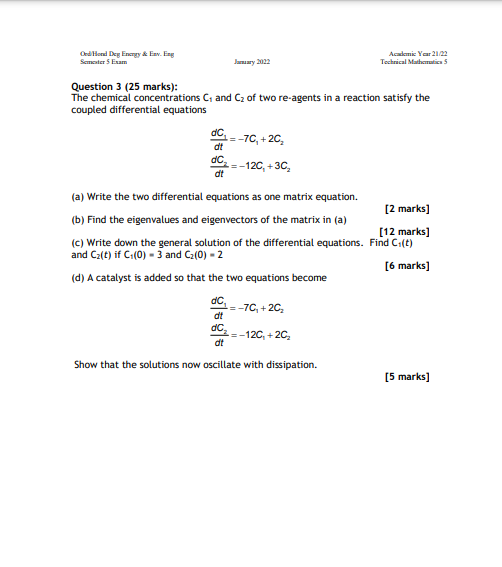# (Solved): PLEASE ANSWER ALL QUESTIONS WITH CLEAR STEPS AND EXPLANATIONS Question 3 (25 marks): The chemical ...Question 3 (25 marks): The chemical concentrations $$C_{1}$$ and $$C_{2}$$ of two re-agents in a reaction satisfy the coupled differential equations $\begin{array}{l} \frac{d C_{1}}{d t}=-7 C_{1}+2 C_{2} \\ \frac{d C_{2}}{d t}=-12 C_{1}+3 C_{2} \end{array}$ (a) Write the two differential equations as one matrix equation. (b) Find the eigenvalues and eigenvectors of the matrix in (a) [2 marks] (c) Write down the general solution of the differential equations. and $$C_{2}(t)$$ if $$C_{1}(0)=3$$ and $$C_{2}(0)=2$$ (d) A catalyst is added so that the two equations become [6 marks] $\begin{array}{l} \frac{d C_{1}}{d t}=-7 C_{1}+2 C_{2} \\ \frac{d C_{2}}{d t}=-12 C_{1}+2 C_{2} \end{array}$ Show that the solutions now oscillate with dissipation.

We have an Answer from Expert